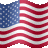# Time Series Projection Trend for ThinkorSwim#### J007RMC

##### Active member
2019 Donor
VIP
Mobius: Here's a study that uses an old method of predicting what price is likely to do in the very short term. The study uses linear regression as the forecasting tool. It looks at the current trend over a short period and a period one magnitude higher adds the slope of those trends then differences that with price to plot an oscillator around 0. If both periods agree the probability is trend will continue for one standard deviation of the shorter n period or n * .68. I added the arrows as overlay.
Code:
``````plot Data = close;# Time Series Projection
# Mobius
# V01.2018
# Uses Linear Regression to predict the price direction. Momentum suggests that the path of least resistance is determined by recent price trend. This indicator uses an order of magnitude in differencing a near and longer term projection.
# Use: When both near and long term agree - Probability is in that the trend will maintain current direction for (n * .68) bars.

declare lower;
input n = 5;

def n2 = n * 10;
def c = close;
def LRL = inertia(c, n);
def slope = AbsValue(LRL - LRL[n]) / n;
def SP = LRL + slope * floor(sqrt(n));
def LRL2 = inertia(c, n2);
def slope2 = AbsValue(LRL - LRL[n2]) / n2;
def SP2 = LRL2 + slope2 * floor(sqrt(n2));
plot OSC = (c - SP) / c;
OSC.HideBubble();
OSC.HideTitle();
plot OSC2 = (c - SP2) / c;
OSC2.HideBubble();
OSC2.HideTitle();
plot ZeroLine = 0;
ZeroLine.HideBubble();
ZeroLine.HideTitle();
Addlabel(if (OSC < 0 and OSC2 < 0)
or (OSC > 0 and OSC2 > 0)
then 1
else 0,
"Prob Trend Cont",
if OSC > 0 then color.green else color.red);
# End Code``````

Last edited by a moderator:
•MerryDay
K

#### KarlWolff

##### Member
Thanks a lot!! This is a great addition to my charts.

•whats up and J007RMC
T

#### Tostechnical

##### Member
@J007RMC This indicator looks very interesting. Could you explain it a little more? Thanks!#### J007RMC

##### Active member
2019 Donor
VIP
Its based on linear regression Ill repost the info I have here
Mobius: Here's a study that uses an old method of predicting what price is likely to do in the very short term. The study uses linear regression as the forecasting tool. It looks at the current trend over a short period and a period one magnitude higher adds the slope of those trends then differences that with price to plot an oscillator around 0. If both periods agree the probability is trend will continue for one standard deviation of the shorter n period or n * .68. I added the arrows as overlay.

T

#### Tostechnical

##### Member
@J007RMC Let me make sure I understand this correctly. So Osc looks for the current trend in a short period using linear regression of the price. So on a daily chart a few days. While OSC2 is the same as OSC except that it is a longer length and uses the slope of the linear regression, used in OSC. If both are above 0 then a positive trend and if both below zero then its a negative trend. Also not a momentum oscillator. Did i misinterpret anything?

K

#### Kimberly

##### New member

When I installed the script, the result looks like this. What am I doing wrong? Thank you.

T

##### Active member
2019 Donor
i tried as well its not working

T

##### Member
VIP
@Kimberly

Just delete plot Data = close; from your first line of code so that the first line reads # Time Series Projection

K

#### Kimberly

##### New member
@Kimberly

Just delete plot Data = close; from your first line of code so that the first line reads # Time Series Projection

•Leavitt Projection Indicator for ThinkorSwim Indicators 10